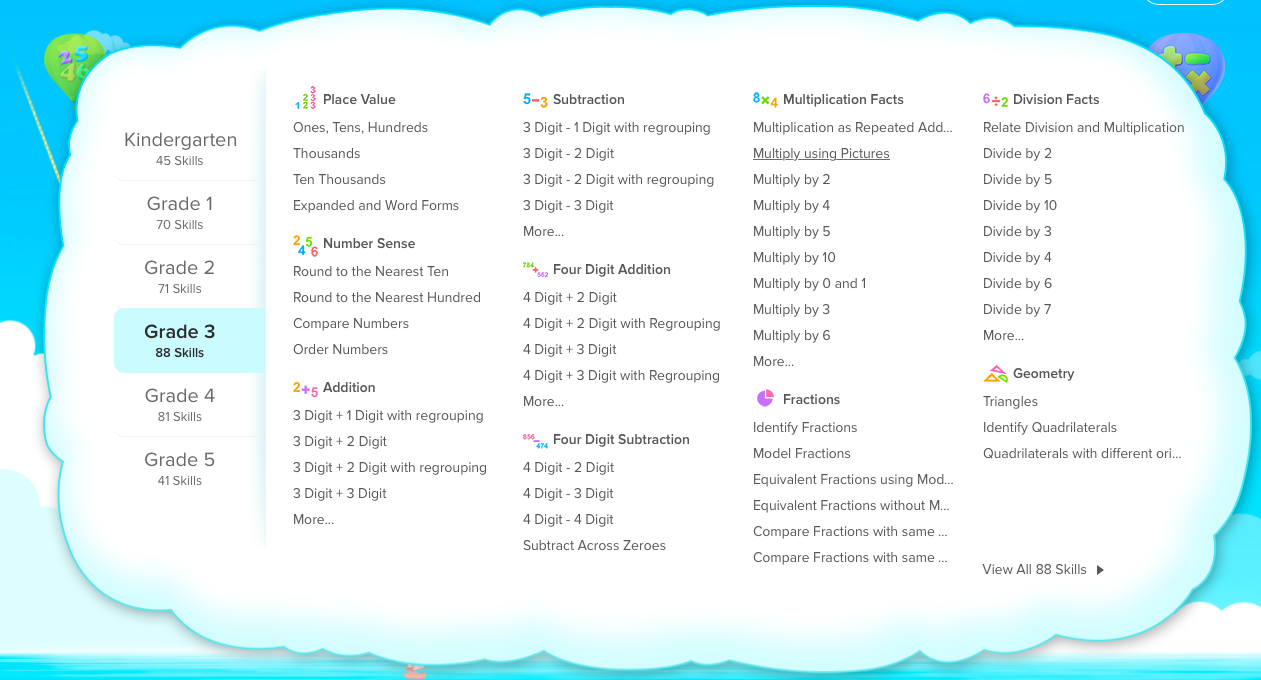# Splash Math Curriculum Structure

Splash Math Curriculum is structured on the basis of the following principles:

1. Standards
3. Challenge
4. Progression
5. Relevance
6. Fun & Engagement

Splash Math provides comprehensive math curriculum for kids from Kindergarten through Grade 5. From time to time, Splash Math reviews existing curriculum and includes new math skills to better the pedagogical objectives and learning experience.The Splash Math curriculum is divided into 6-grade levels starting from Kindergarten to Grade 5. Each student can practice across grade-levels depending on their current math proficiency level and the purchased Splash Math plan.

Topics

Each grade level is further sub-divided into topics or chapters. For example - Grade 3 Curriculum is divided into the following topics as seen in the image:

• Place Value
• Number Sense
• Subtraction
• Four Digit Subtraction
• Multiplication Facts
• Fractions
• Division Facts
• Geometry

Skills

Each topic is further sub-divided into skills. A skill is typically a singular math concept which is introduced in a single class by the teacher. A teacher can introduce the skill in the class and then have students practice them on Splash Math.

Levels

Splash Math Skills are further broken down into multiple levels based on the progression of difficulty level and different learning strategies. For example: a one digit addition skill could include problems on the following levels -

1. One Digit Addition with pictures
2. One Digit Addition with base-ten blocks
3. Numbers < 5
4. Numbers < 7
5. Numbers <= 9
6. Without Carry
7. With Carry
8. Missing number problems
9. Word Problems